# RD Sharma Solutions Class 6 Maths Chapter 14 Circles

Read RD Sharma Solutions Class 6 Maths Chapter 14 Circles below, students should study RD Sharma class 6 Mathematics available on Studiestoday.com with solved questions and answers. These chapter wise answers for class 6 Mathematics have been prepared by teacher of Grade 6. These RD Sharma class 6 Solutions have been designed as per the latest NCERT syllabus for class 6 and if practiced thoroughly can help you to score good marks in standard 6 Mathematics class tests and examinations

Exercise 14.1

Question 1:  Explain the following:

(i) Circle

(iii) Centre

(iv) Diameter

(v) Chord

(vi) Interior of a circle.

Solution 1:

(i) Circle:-  A circle is a simple closed curve which is not a polygon. It is a set of all those points in a plane whose distance is equal from a fixed point. For Example:- a wheel, a bangle, a coin etc.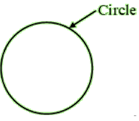(ii) Radius:-   The distance between the all the points of the circle to its centre is called radius.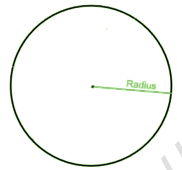(iii) Centre:–  The mid-point of a circle which is at a constant distance from all the points called centre.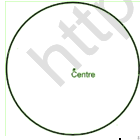(iv) Diameter:–  Diameter is a line segment passing through the centre of a circle, and having its end-points on the circle.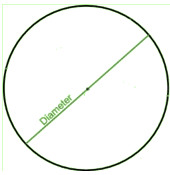(v) Chord:–  Chord of a line segment with its end-points lying on a circle.(vi) Interior of a circle:–

The inside plane part of a circle called the interior of a circle.

Question 2:   Take a point on your notebook and draw circle of radii 4 cm, 3 cm and 6.5 cm, each having the same centre O.

Solution 2:    The figure given below shows circles of 4 cm, 3 cm and 6.5 cm radii having the same centre.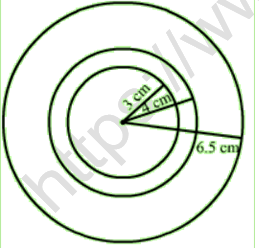Question 3:  Draw a circle with centre O and any radius. Draw AC and BD two perpendicular diameters of the circle. Join AB, BC, CD and DA.

Solution 3: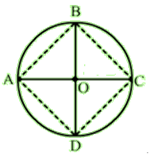Question 4:  Draw a circle with centre O and radius 6 cm. Mark points P, Q, R such that

(i) P lies on the circle,

(ii) Q lies in the interior of the circle, and

(iii) R lies in the exterior of the circle.

Rewrite each of the following statements using the correct symbol (=, < or >):

(i) OQ …… 5 cm (ii) OP ……. 5 cm (iii) OR …… 5 cm.

Solution 4: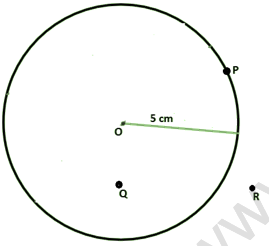The statements can be written as
(i) OQ < 5 cm OQ is smaller than 5.
(ii) OP = 5 cm OP is Equal to 5.
(iii) OR > 5 cm OR is greater than 5.

Question 5:   Take two points A and B on the page of your note book. Draw a circle with centre A which passes through B.

Solution 5:Question 6:  Draw a semi-circle with centre O and radius 5 cm. Is the diameter that determines the semi-circle, a part of the semi-circle?

Solution 6:   The figure given below shows a semi-circle with centre O and radius 5 cm.The end point of the diameter of a circle divides the circle into equal parts. The diameter does not determine the semi-circle and it is the end points of the diameter which finds the semi-circle or a part of the semi-circle.

Question 7:  The diameter of a circle is 14 cm, find its radius.

Solution 7:   Diameter of a circle = 14 cm

Hence, the Radius of a circle is 7cm.

Question 8:  Given a circle with centre O and radius 2.5 cm, what is the length of the longest chord of the circle.

Solution 8:

The diameter of a circle is diameter.

Diameter = 2 × 2.5

Diameter = 5 cm

Hence, the length of the longest chord of the circle is 5cm.

Question 9:  Fill in the blanks:

(i) The diameter of a circle is ___________ times its radius.

(ii) The diameter of a circle is the ___________ chord of the circle.

(iii) The diameter of a circle pass through ___________.

(iv) A chord of a circle is a line segment with its end points on the ___________

(v) If we join any two points on a circle by a line segment, we obtain ___________ of the circle.

(vi) A radius of a circle is a line segment with one end at ___________ and the other end at _________.

(vii) All radii of a circle are ___________.

(viii) The diameters of a circle are ___________.

(ix) The total number of diameters of a circle is ___________.

(x) Every point on a circle is ___________ from its centre.

(xi) A chord of a circle contains exactly ___________ points of the circle.

(xii) A diameter is the longest ___________.

(xiii) Concentric circles are circles having ___________.

Solution 9:

(i) The diameter of a circle is two times its radius.

(ii) The diameter of a circle is the longest chord of the circle.

(iii) The diameter of a circle pass through its centre.

(iv) A chord of a circle is a line segment with its end points on the circle.

(v) If we join any two points on a circle by a line segment, we obtain chord of the circle.

(vi) A radius of a circle is a line segment with one end at centre and the other end at circle.

(vii) All radii of a circle are equal.

(viii) The diameters of a circle are concurrent.

(ix) The total number of diameters of a circle is infinite.

(x) Every point on a circle is equidistant from its centre.

(xi) A chord of a circle contains exactly two points of the circle.

(xii) A diameter is the longest chord.

(xiii) Concentric circles are circles having same centre.

Question 10:  In each of the following, state if the statement is true (T) or false (F):
(i) Every circle has a centre.
(ii) The centre of a circle is a point of the circle.
(iii) Any two radii of a circle make up a diameter.
(iv) Every chord of a circle is parallel to some diameter of the circle.
(v) A circle is symmetric about each of its diameters.
(vi) The diameter is twice the radius.
(vii) A radius is a chord of the circle.
(viii) Concentric circles have the same radii.
(ix) The nearer a chord to the centre of a circle, the longer is its length.

Solution 10:
(i) Every circle has a centre. True
(ii) The centre of a circle is a point of the circle. False
(iii) Any two radii of a circle make up a diameter. False
(iv) Every chord of a circle is parallel to some diameter of the circle. False
(v) A circle is symmetric about each of its diameters. True
(vi) The diameter is twice the radius. True
(vii) A radius is a chord of the circle. False
(viii) Concentric circles have the same radii. False
(ix) The nearer a chord to the centre of a circle, the longer is its length. True

Objective Type Questions ::->

Mark the correct alternative in each of the following:

Question 1:  A circle of radius r cm has diameter of length

(a) r cm

(b) 2r cm

(c) 4r cm

(d) r/2 cm

Solution 1: (b)

D = 2r

Question 2:  A chord of a circle passing through its centre is equal to its

(b) diameter

(c) circumference

(d) none of these

Solution 2: (b)

Diameter:-  A chord of a circle passing through its centre.

Question 3: The total number of diameters of a circle is

(a) 1

(b) 2

(c) 4

(d) uncountable number

Solution 3: (d)

Many diameters can be formed in circle.

Question 4: By joining any two points on a circle, we obtain its

(b) diameter

(c) chord

(d) circumference

Solution 4: (c)

We can obtain chord by joining any two points on a circle.

Question 5:  The longest chord of a circle is equal to its

(b) diameter

(c) circumference

(d) perimeter

Solution 5: (b)

Diameter:- The longest chord of a circle.

Question 6:  How many circles can be drawn to pass through two given points?

(a) 1

(b) 2

(c) 0

(d) As many as possible

Solution 6: (d)

As many as possible circles can be drawn to pass through two given points.

Question 7:  How many circles can be drawn to pass through three non-collinear points?

(a) 1

(b) 2

(c) 0

(d) As many as possible

Solution 7: (a)

By three non-collinear points we can draw only 1 circle.